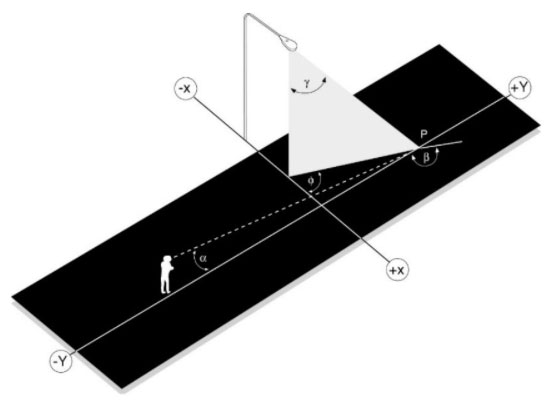# reduced luminance coefficient, r

[11.5.20]

The luminance coefficient, q, multiplied by the cube of the cosine of the angle of incidence, (cos γ)3, of the light on the point (see figure):

r = q(cos γ)3 ,

where:

q is the luminance coefficient in sr-1

γis the angle of incidence in degrees (the road surface is flat and not sloped up-down or right-left)

Unit: sr-1

Note 1: The angle of observation α, affects the value of r. By convention, this angle is fixed at 1° for roadway lighting calculations.

Note 2: The reduced luminance coefficient is used for determining the luminance of a point of infinitesimal size in the roadway luminance formula.